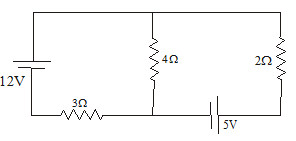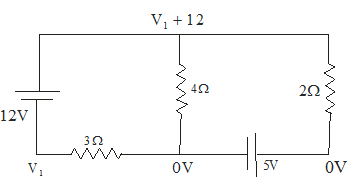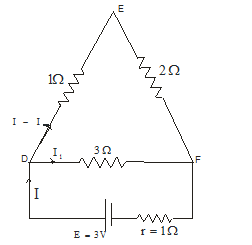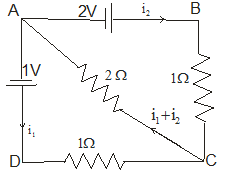# Problems on electric Circuits

Question 1
A circuit is shown in below figure(a)Find the current in the 3Ω,4Ω,2 Ω
(b) What is the PD across 4 Ω resistors

Consider the negative terminal of the 5 V battery is at zero volt and then we can write the potential of other nodesApply Kirchhoff rule of junction at the node having potential (V1 +12) Volt
$\frac {V_1 +12 -0}{4} + \frac {V_1 -0}{3} + \frac {V_1 +12 -5}{2} =0$
Or V1=-6V
So current in 4 Ω=(V1+12)/4=1.5 A
Current in 3 Ω=6/3=2A
Current  in 2 Ω=(6-5)/2=.5 A
Potential difference across 4 Ω=6V

Question 2
Three resistors are joined to form a triangle DEF such that DE=1 Ω, EF=2 Ω and FD=3 Ω.A battery of EMF 2 V and internal resistance 1 Ω is connected to points FD
(a)Find the current flowing in DF and DE side
(b)Find the Potential difference at the battery terminals
(c)Find the Potential difference along the DE arm

Three resistors of 1 Ω,2 Ω,3 Ω are connected to form a triangle DEF as shown in below figure.Since the resistance along DEF are in series
So R=1+2=3 Ω
Now DEF is parallel to DF
So Net resistance
$R= \frac {3X3}{3+3} = 1.5 \Omega$
Let I be the current in the battery then
We know that
$I = \frac {E} {r+R}$
So
$I=\frac {3} {1+1.5} =1.2 A Let I1 current flows along the path DF so I-I1 current will flow in the path DEF PD across DF=I1*3 PD across DEF=(I-I1)*3 Since PD will be same across the both I1=I/2=.6 A So the current along DF=.6 A And current across DEF=.6 A Potential difference across the cell = I1*3 =.6*3=1.8 V Potential difference along the DE = I1*1=.6 V Question 3 A galvanometer together with a resistance 2 Ω is series is connected across two identical batteries each of 1.5 V.When the batteries are connected in series ,the galvanometer records a current of 1 A .When the batteries are connected in parallel, the current is .6 A. (a)Find the internal resistance of the battery (b) Find the resistance of the Galvanometer Answer Let r be the internal resistance of the battery. Let G be the resistance of the galvanometer. Let E be the emf of each battery When the batteries are connected in series Total EMF=2E and Total internal resistance=2r So the current in the circuit will be$I =\frac {3} {G+2+2r}$Given I=1A Therefore$1=\frac {3} {G+2+2r}$G+2=(3-2r) ---(1) When the batteries are connected in parallel, the total emf E=1.5V and the total internal resistance =r/2.Hence the current in the circuit will be$I^' =\frac {1.5}{G+2+ \frac {r}{2} } $Given I=.6 A Therefore$.6= \frac {1.5}{G+2+ \frac {r}{2} } $Or G+2=(2.5-r/2) --(2) From equation 1 and 2 3-2r=2.5-r/2 Or r=1/3 Ω Now from equation 1 G+2=3-(2/3) G=1-(2/3) =1/3 Ω Question 4 A battery of EMF 10V is connected with three resistors A, B, C having resistance 1, 2, 3 ohms respectively in series. The voltage across the resistor B is measured by a voltmeter whose resistance is 10 ohm. What is the percentage error? Answer The Total resistance of the circuit before connecting the voltmeter =1+2+3=6 ohm The current in the circuit would be I=10/6 The voltage across B resistors will be =iR= (10/6)*2=10/3 This is the voltage to be measured Now when the voltmeter is connected across the resistor B,The voltage will be parallel to the resistor .Since the voltmeter has internal résistance, The effective resistance across the resistors B = 2*10/(2+10)=5/3 Total resistance to the battery=1+5/3+3=17/3 Current =3E/17 Now voltage across the resistor B =iR=(3E/17)(5/3)=5E/17=50/17 This will be the reading in the voltmeter The error in reading =(10/3) –(50/17) =20/51 % error in reading=$\frac {20/51}{10/3} X 100 $=11.76% Question 5 Two batteries each of EMF E and internal resistance r are connected in parallel. If we take current from the combination in an external resistance R, (a) Find the power dissipated in the resistance R (b) What value of R maximum power will be obtained? (c) What is the value of maximum power? Answer From parallel group of cell we know that$E=\frac {E_1 r_1 + E_2 r_2}{r_1 + r+2} =E r= \frac {r_1 r_2}{r_1 + r_2} = r/2 $Now current$I= \frac {E}{ \frac {r}{2} + R} = \frac {2E}{ r+2R}$Power dissipated in the Resistance R=i2R$=\frac {(2E)^2 R}{ (r+2R)^2} = \frac {4E^2 R}{(r-2R)^2 + 8rR}$For Power to be maximum the denominator should be minimum i.e (r-2R)2=0 R=r/2 Therefore,$P_max = \frac {4E^2 (r/2)}{(r+r)^2}=\frac {E^2}{2r}\$

Question 6
An electrical circuit is shown in the below figure. The internal resistance of the cells is negligible. Find the current in the each resistorLet i1 and i2 be the current flowing from the cells of 1 and 2 volt respectively.
Applying the K rchhoff‘s first law at junction C ,the current in the 2 Ω will be i1+i2
Applying the Kirchhoff second law of closed mesh ADCA ,we have
i1*1+(i1+i2)*2=1
3i1+2i2=1     -(1)
Similarly for the closed mesh ABCA,we have
i2*1+(i1+i2)*2=2
2i1+3i2=2     -(2)
Solving equation  (1) and (2),we have
I1=-.2A
I2=.8 A
The current in the 2 Ω resistors = i1+i2=.6 A

Question 7
The potential difference across a cell is 1.8 V when a current of .5 A is drawn from it. The PD falls to 1.6 V volt when a current of 1.0 A is drawn .Find the EMF and internal resistance of the cell

Suppose the EMF of the cell is E and internal resistance is r
Now we know
V=E-iR
When i=.5 A V=1.8
1.8=E-.5r     -(1)
When i=1, V=1.6
1.6=E-r --- (2)
Solving equation (1) and (2)
E=2 volt and r=.4  ohm

Question 8
What is the resistance of a 1000W 120 V toaster?

Power is given by
P=VI
Given P=1000 and V=120
So 1000=120I
Or I=8.333 A
Now from Ohm’s law
V=IR
Or R=V/I
=14.4 ohm

Question 9
A 600 W heater is used to heat .26 kg of water from 293K to 373 K.What is minimum time in which this can be done?
Specific heat of water=4.184 KJ/kg.K

The electric energy generated is given by
=Pt
Given P=600 W
So EE=600t=.600t KJ
The heat required to warm the water
=mc =.26*4.184*80=87.02 kJ
Now
Heat required to warm up the water=Electric energy generated by the heater
.600t=87.02
Or t=145 sec